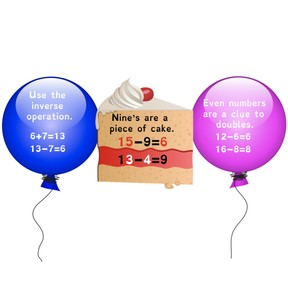Automaticity subtraction to 20

# Automaticity subtraction to 20

I can solve subtraction facts to 10 and 20.

No account needed.8,000 schools use Gynzy92,000 teachers use Gynzy1,600,000 students use Gynzy

## General

Students will practice automaticity with subtraction facts to 20. The lesson incorporates the use of the inverse operation of addition, using halves, and subtraction with 9’s.

## Standards

CCSS.Math.Content.2.OA.B.2

## Learning objective

Students will be able to solve subtraction facts to 10 and 20.

## Introduction

Students will review the idea of thinking mentally. They listen to a shopping list of 5 items and then they try to pick out the objects from a larger group of twelve things. This activity helps them to recognize the importance of thinking mentally and how that applies to memorizing math facts.

## Instruction

Using the Gynzy problem generator, students will review the facts with a minuend of10 or less. This is followed by a discussion of using the inverse property of addition to recalling facts they already are familiar with. The students practice solving facts, then saying the inverse operation aloud. They check by listening to the answers. Next, the doubles addition facts are related to halving an even number when you subtract. Techniques of working with 9’s are covered with opportunities to practice. The lesson continues with a timed fact drill and timed flashcards.

## Quiz

Students respond to ten multiple-choice and true/false questions.

## Closing

Students will complete a Gynzy matching game that uses pairs of facts that demonstrate the inverse operation.

### The online teaching platform for interactive whiteboards and displays in schools

• Save time building lessons

• Manage the classroom more efficiently

• Increase student engagement